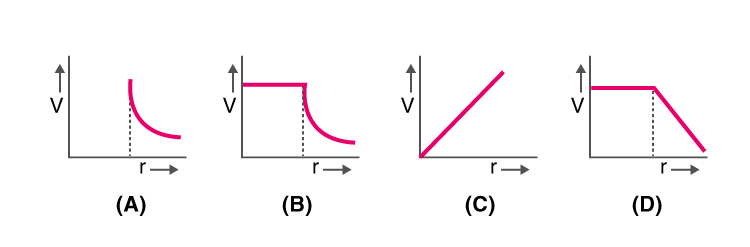## Physics MCQ on Electro statistics for NEET, JEE, Medical and Engineering Exam 2022

MCQ on Electro statistics: Previous trends reveal that NEET/JEE aspirants find Physics as the most challenging section amongst the other sections. But most of them fail to decipher the reason behind this. This particularly can be as the candidates taking up this examination are predominantly medical aspirants with their subject of interest being Biology. Also, sections like Physics are more on theories, laws, numerical as opposed to Biology which is more of fact-based, life sciences and comprising of substantial explanations.In this post we are providing you MCQ on Electro statistics, which will be beneficial for you in upcoming NEET, AIIMS, JEE, WBJEE, Medical and Engineering Exams.

## MCQ on Electro statistics

Q1. What happens to the force acting between the charged particles, if the distance between these charged particles is halved?
a) It increases by four times
b) It gets doubled
c) It becomes half
d) It reduces by one-fourth

(a) It increases by four times

Q2. The capacitance of the capacitor is independent of
a) The charges present on the plate
b) The distance of separation between the plates
c) The shape of the plates
d) The size of the plates

(d) The size of the plates

Q3. For a hollow spherical shell, potential, V changes with respect to distance, r from the centre. Which graph from the following represents this?(b)

Q4. What will be the flux coming out of any surface a cube, if a change QμC is placed at the centre of the cube?
a) Q/6ϵ0×10^3
b) Q/24ϵ0
c) Q/8ϵ0
d) Q/6ϵ0×10^−6

(d) Q/6ϵ0×10^6

Q5. The mathematical representation of the force of attraction between the plates of the parallel plate capacitor is given as
a) q²/2ϵ0K
b) q²/ϵ0AK
c) q²/2ϵ0AK
d) q²/2ϵ0A

(c) q²/2ϵ0AK

Q6. What is the electric potential of the points A, B, and C which are in a uniform electric field?a) The electric field is the same at all three points A, B, and C
b) Maximum at A
c) Maximum at B
d) Maximum at C

(c) Maximum at B

Q7. Name the law that states the force between electric charges
a) Ohm’s law
b) Coulomb’s law
d) Ampere’s law

(b) Coulomb’s law

Q8. The capacity of parallel plate condenser is dependent on the
a) The separation between the plates
b) The metal used for the construction
c) The thickness of the plate
d) The potential applied across the plates

(a) The separation between the plates

Q9. What is the minimum charge on a particle?
a) 1 coulomb
b) 1.6 x 10-19 Coulomb
c)  3.2x 10-19 Coulomb
d) 6.6x 10-19 Coulomb

(b) 1.6 x 10-19 Coulomb

Q10. Consider two capacitances of capacity C1 and C2 which are connected in series and have potential difference V. What is the potential difference across C1?
a) V(C1/C1+C2)
b) V(C1+C2/C1)
c) V(C1/C2)
d) V(C2/C1+C2)

(d) V(C2/C1+C2)

Q11. What does an electric dipole experience when it is kept in the non-uniform electric field?
a) Only a force
b) Only a torque
c) Force and torque both
d) Neither force nor torque

(c) Force and torque both